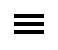Course Details

# High Impact Excel: LookUp FormulasWebinar: ID# 1015359
Recorded On-Demand
Qty:
Look-up formulas add automation to Excel spreadsheets in that users are freed from having to manually point to a designated cell that contains text or numbers. For instance, you might use look-up formulas to return the price for an inventory item, or use SUMIF or SUMIFS to summarize data based upon one or more criteria.

In this session Excel expert David Ringstrom introduces beginners to the VLOOKUP function, and then goes beyond the basics with a discussion of the MATCH, CHOOSE, INDEX, SUMIF, SUMIFS, and SUMPRODUCT functions.

David's presentations are fast-paced, so even seasoned Excel users are likely to learn several new tricks and techniques. David will teach primarily from Excel 2010 but will cover differences in Excel 2003, 2007, and 2013 where applicable.

Learning Objectives:
• Understand VLOOKUP and its nuances and restrictions
• Explore alternatives to VLOOKUP, such as MATCH/INDEX, SUMIF, and SUMIFS.
• Avoid common pitfalls that plague users of look-up formulas
• Improve the integrity of spreadsheets with Excel's VLOOKUP function
• Future-proof VLOOKUP by using Excel's Table feature instead of referencing static ranges
• Perform dual lookups, where you look across columns and down rows to cross reference the data you need
• See how to arrange two worksheets from within the same workbook onscreen at the same time
• Discover how to use wildcards and multiple criteria within look-up formulas
• Uncover the limitations of VLOOKUP, along with alternatives
• Avoid the complexity of nested IF statements with Excel's CHOOSE function
• See why the MATCH and INDEX combination is often superior to VLOOKUP or HLOOKUP
• Master the versatile SUMIF function
• Use the SUMIFS to sum value based on multiple criteria
• See the variety of functionality the SUMPRODUCT function offers
• Compare and contrast IFNA, IFERROR, and ISERROR, and see which versions of Excel support each of these worksheet functions
Level: Basic
Prerequisites: NoneCPATrainingCenter.com. 5755 North Point Parkway, Suite 227 | Alpharetta, GA 30022 | 770-410-9375 | support@CPATrainingCenter.com